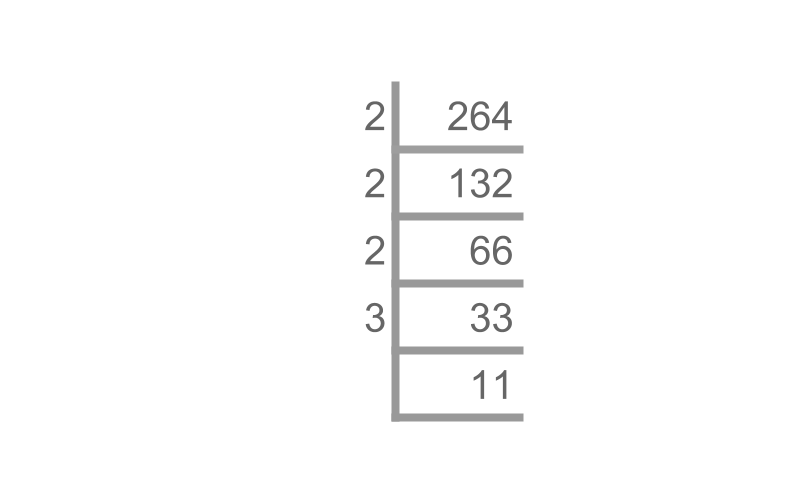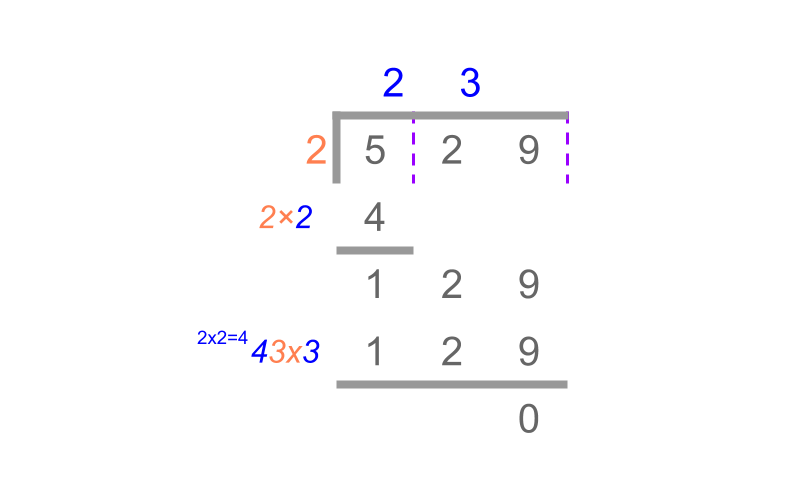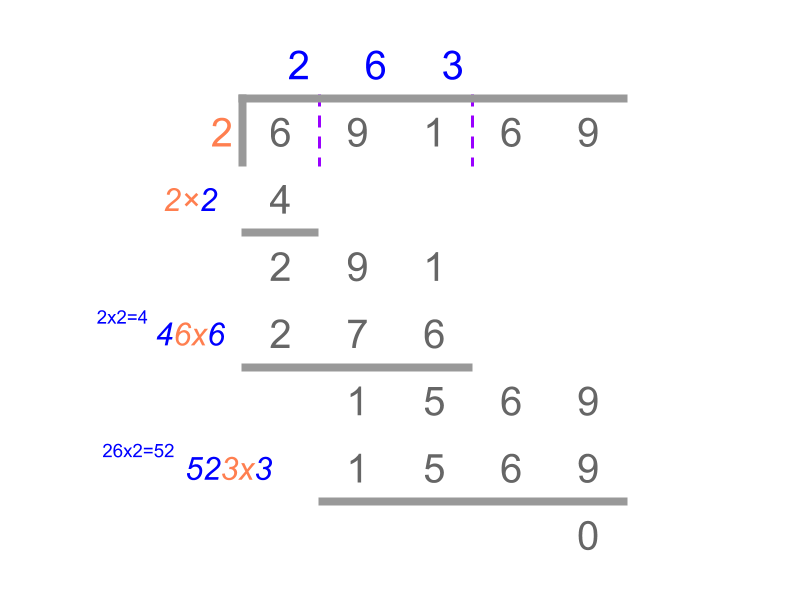maths > exponents

Representation of Squares and Square Roots

what you'll learn...

overview

In this page, square of a number and square root of a number are revised. The long division method to find the square root is explained.

square

We studied in exponents that ${3}^{2}=3×3=9$${3}^{2} = 3 \times 3 = 9$.

An exponent of power $2$$2$ is called square of the number.

eg: Square of $7$$7$ is ${7}^{2}=49$${7}^{2} = 49$.

The word "square" means : the 2D shape of equal sides and ${90}^{\circ }$${90}^{\circ}$ angles" between the sides. The exponent square is representative of "area of the shape square, side to the power $2$$2$".

root

We studied in the exponents that ${16}^{\frac{1}{2}}=\sqrt{16}=4$${16}^{\frac{1}{2}} = \sqrt{16} = 4$".

An exponent of power $\frac{1}{2}$$\frac{1}{2}$ or the $2$$2$nd root is called square root of the number.

eg: Square root of $64$$64$ is ${64}^{\frac{1}{2}}=\sqrt{64}=8$${64}^{\frac{1}{2}} = \sqrt{64} = 8$.

The power of the root need not be mentioned for square roots
$\sqrt{64}=\sqrt{64}$$\sqrt{64} = \sqrt{64}$.

examples

Square of $4$$4$ is ${4}^{2}$${4}^{2}$.

Square root of $9$$9$ is $\sqrt{9}={9}^{\frac{1}{2}}$$\sqrt{9} = {9}^{\frac{1}{2}}$.

$\sqrt{100}={100}^{\frac{1}{2}}=10$$\sqrt{100} = {100}^{\frac{1}{2}} = 10$".

the symbol $\sqrt{}$$\sqrt{}$ is the square root.

definitions

Square of a number : A number multiplied by itself is the square of the number.
eg: ${6}^{2}=6×6=36$${6}^{2} = 6 \times 6 = 36$

Square Root of a number : Square root of a value is the number whose square is the given value.
eg: $\sqrt{36}=6$$\sqrt{36} = 6$ as ${6}^{2}=36$${6}^{2} = 36$.

factorize

The factors of $26$$26$ are "$1,2,13,26$$1 , 2 , 13 , 26$"

The factors of $28$$28$ are "$1,2,4,7,14,28$$1 , 2 , 4 , 7 , 14 , 28$"The prime factorization of $264$$264$ is $2×2×2×3×11$$2 \times 2 \times 2 \times 3 \times 11$.
A procedure is illustrated in the figure.

finding square roots

Consider finding the result of $\sqrt{3600}$$\sqrt{3600}$.

Square root is a form of root. In roots, we learned to perform prime factorization to find the root.
$\sqrt{3600}$$\sqrt{3600}$
$=\sqrt{2×2×2×2×3×3×5×5}$$= \sqrt{2 \times 2 \times 2 \times 2 \times 3 \times 3 \times 5 \times 5}$
$=2×2×3×5$$= 2 \times 2 \times 3 \times 5$
$=60$$= 60$

Finding Square Roots : To find square root of a number, express the number in prime factors and group the factors.

eg: $\sqrt{100}$$\sqrt{100}$ $=\sqrt{2×2×5×5}$$= \sqrt{2 \times 2 \times 5 \times 5}$ $=2×5=10$$= 2 \times 5 = 10$

Note: This method is suitable for finding square roots resulting in integers.

example

What is the $\sqrt{8}$$\sqrt{8}$
The answer is "$2\sqrt{2}$$2 \sqrt{2}$".

Using the prime factorization method
$\sqrt{8}$$\sqrt{8}$
$=\sqrt{2×2×2}$$= \sqrt{2 \times 2 \times 2}$
$=2×\sqrt{2}$$= 2 \times \sqrt{2}$

The prime factorization method is suitable for square roots resulting in integer values.

a procedure

Square root of a number can be given as
${x}^{2}={\left(10a+b\right)}^{2}$${x}^{2} = {\left(10 a + b\right)}^{2}$
${x}^{2}=100{a}^{2}+\left(20ab\right)+{b}^{2}$${x}^{2} = 100 {a}^{2} + \left(20 a b\right) + {b}^{2}$

Note:
${b}^{2}$${b}^{2}$ is a $2$$2$ digit number with tens-units places
$100{a}^{2}$$100 {a}^{2}$ is a number that has $00$$00$ at tens-units places

This understanding gives a method to eliminate $b$$b$ and look at $a$$a$ to choose the highest digit of the square root.

${x}^{2}=100{a}^{2}+\left(20a+b\right)×b$${x}^{2} = \textcolor{c \mathmr{and} a l}{100 {a}^{2}} + \textcolor{\mathrm{de} e p s k y b l u e}{\left(20 a + b\right) \times b}$
${x}^{2}-100{a}^{2}=\left(20a+b\right)×b$${x}^{2} \textcolor{c \mathmr{and} a l}{- 100 {a}^{2}} = \textcolor{\mathrm{de} e p s k y b l u e}{\left(20 a + b\right) \times b}$

The rearranged one with $\left(20a+b\right)$$\left(20 a + b\right)$ gives the method to multiply $a$$a$ by $2$$2$ (which is $2a$$2 a$) and append a value $b$$b$, which is $20a+b$$20 a + b$. Then multiply $b$$b$ to $20a+b$$20 a + b$.

step 1
${x}^{2}-{a}^{2}\phantom{\rule{1ex}{0ex}}\text{at 100s place}=y$${x}^{2} \textcolor{c \mathmr{and} a l}{- {a}^{2} \textrm{a t 100 s p l a c e}} = y$
step 2
$y-\left(2a\phantom{\rule{1ex}{0ex}}\text{joined with}\phantom{\rule{1ex}{0ex}}b\right)×b$$y - \textcolor{\mathrm{de} e p s k y b l u e}{\left(2 a \textrm{j o \in e d w i t h} b\right) \times b}$

In this process, the choice of $a$$a$ and $b$$b$ make the square root $x=10a+b=a\phantom{\rule{1ex}{0ex}}\text{joined with}\phantom{\rule{1ex}{0ex}}b$$x = 10 a + b = a \textrm{j o \in e d w i t h} b$

The above process is explained for 2 digit square root and is easily extended for higher number of digits.

example using the procedureConsider $\sqrt{529}$$\sqrt{529}$

$529$$529$
$={23}^{2}$$= {23}^{2}$
$={\left(10×2+3\right)}^{2}$$= {\left(10 \times 2 + 3\right)}^{2}$
$={\left(10×2\right)}^{2}+2×10×2×3+{3}^{2}$$= {\left(10 \times 2\right)}^{2} + 2 \times 10 \times 2 \times 3 + {3}^{2}$
$=100×{2}^{2}+\left(2×2×10+3\right)×3$$= \textcolor{c \mathmr{and} a l}{100 \times {2}^{2}} + \textcolor{\mathrm{de} e p s k y b l u e}{\left(2 \times 2 \times 10 + 3\right) \times 3}$
$={2}^{2}\phantom{\rule{1ex}{0ex}}\text{100s place}\phantom{\rule{1ex}{0ex}}+\left(2×2\phantom{\rule{1ex}{0ex}}\text{joined}\phantom{\rule{1ex}{0ex}}3=43\right)×3$$= \textcolor{c \mathmr{and} a l}{{2}^{2} \textrm{100 s p l a c e}} + \textcolor{\mathrm{de} e p s k y b l u e}{\left(2 \times 2 \textrm{j o \in e d} 3 = 43\right) \times 3}$

The above steps is used in reverse when the square root is not known
$\sqrt{529}$$\sqrt{529}$
$=2\phantom{\rule{1ex}{0ex}}\text{at 10s place}\phantom{\rule{1ex}{0ex}}+3\phantom{\rule{1ex}{0ex}}\text{at units place}$$= \textcolor{c \mathmr{and} a l}{2} \textrm{a t 10 s p l a c e} + \textcolor{\mathrm{de} e p s k y b l u e}{3} \textrm{a t u n i t s p l a c e}$
$=23$$= 23$

This procedure is illustrated in the figure.

$\sqrt{69169}=263$$\sqrt{69169} = 263$summary

Procedure to finding Square Root of a number : Long division method is illustrated in the figure.Outline

The outline of material to learn "Exponents" is as follows. Note: click here for detailed outline of Exponents s

→   Representation of Exponents

→   Inverse of exponent : root

→   Inverse of exponent : Logarithm

→   Common and Natural Logarithms

→   Exponents Arithmetics

→   Logarithm Arithmetics

→   Formulas

→   Numerical Expressions

→   PEMA / BOMA

→   Squares and Square roots

→   Cubes and Cube roots